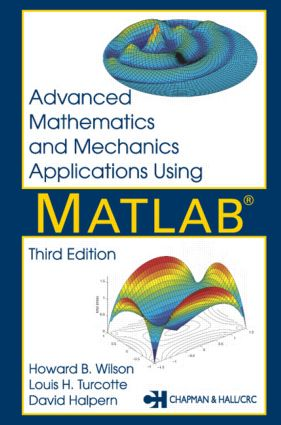Advanced Mathematics and Mechanics Applications Using MATLAB

3rd Edition

Chapman and Hall/CRC

696 pages | 138 B/W Illus.

Purchasing Options:\$ = USD
Hardback: 9781584882626
pub: 2002-09-17
SAVE ~\$34.00
\$170.00
\$136.00
x
eBook (VitalSource) : 9780429122736
pub: 2002-09-17
from \$28.98

FREE Standard Shipping!

Description

Since its introduction in 1984, MATLAB's ever-growing popularity and functionality have secured its position as an industry-standard software package. The user-friendly, interactive environment of MATLAB 6.x, which includes a high-level programming language, versatile graphics capabilities, and abundance of intrinsic functions, helps users focus on their applications rather than on programming errors. MATLAB has now leapt far ahead of FORTRAN as the software of choice for engineering applications.

Reviews

"Material [in this book] can serve as a valuable resource for the AEM course that provides a brief exposure to numerics. It can also supplement the more heavily numerical course."

- SIAM Review, Vol. 46, No. 3

INTRODUCTION

MATLAB: A Tool for Engineering Analysis

Use of MATLAB Commands and Related Reference Materials

Example Problem on Financial Analysis

Computer Code and Results

ELEMENTARY ASPECTS OF MATLAB GRAPHICS

Introduction

Overview of Graphics

Example Comparing Polynomial and Spline Interpolation

Conformal Mapping Example

Nonlinear Motion of a Damped Pendulum

A Linear Vibration Model

Example on Waves in an Elastic String

Properties of Curves and Surfaces

SUMMARY OF CONCEPTS FROM LINEAR ALGEBRA

Introduction

Vectors, Norms, Linear Independence, and Rank

Systems of Linear Equations, Consistency, and Least Square Approximation

Applications of Least Square Approximation

Eigenvalue Problems

Computing Natural Frequencies for a Rectangular Membrane

Column Space, Null Space, Orthonormal Bases, and SVD

Computation Time to Run a MATLAB Program

METHODS FOR INTERPOLATION AND NUMERICAL DIFFERENTIATION

Concepts of Interpolation

Interpolation, Differentiation, and Integration by Cubic Splines

Computing the Length and Area Bounded by a Curve

Numerical Differentiation Using Finite Differences

GAUSS INTEGRATION WITH GEOMETRIC PROPERTY APPLICATIONS

Fundamental Concepts and Intrinsic Integration Tools in MATLAB

Concepts of Gauss Integration

Comparing Results from Gauss Integration and Function QUADL

Geometrical Properties of Areas and Volumes

Computing Solid Properties Using Triangular Surface Elements and Using Symbolic Math

Numerical and Symbolic Results for the Example

Geometrical Properties of a Polyhedron

Evaluating Integrals Having Square Root Type Singularities

Gauss Integration of a Multiple Integral

FOURIER SERIES AND THE FAST FOURIER TRANSFORM

Definitions and Computation of Fourier Coefficients

Some Applications

DYNAMIC RESPONSE OF LINEAR SECOND ORDER SYSTEMS

Solving the Structural Dynamics Equations for Periodic Applied Forces

Direct Integration Methods

INTEGRATION OF NONLINEAR INITIAL VALUE PROBLEMS

General Concepts on Numerical Integration of Nonlinear Matrix Differential Equations

Runge-Kutta Methods and the ODE Integrator Provided in MATLAB

Step-Size Limits Necessary to Maintain Numerical Stability

Discussion of Procedures to Maintain Accuracy by Varying Integration Step-size

Example on Forced Oscillations of an Inverted Pendulum

Dynamics of a Spinning Top

Motion of a Projectile

Example on Dynamics of a Chain with Specified End Motion

Dynamics of an Elastic Chain

BOUNDARY VALUE PROBLEMS FOR LINEAR PARTIAL DIFFERENTIAL EQUATIONS

Several Important Partial Differential Equations

Solving the Laplace Equation Inside a Rectangular Region

The Vibrating String

Force Moving on an Elastic String

Waves in Rectangular or Circular Membranes

Wave Propagation in a Beam with an Impact Moment Applied to One End

Forced Vibration of a Pile Embedded in an Elastic Medium

Transient Heat Conduction in a One-Dimensional Slab

Transient Heat Conduction in a Circular Cylinder with Spatially Varying Boundary Temperature

Torsional Stresses in a Beam of Rectangular Cross Section

EIGENVALUE PROBLEMS AND APPLICATIONS

Introduction

Approximation Accuracy in a Simple Eigenvalue Problem

Stress Transformation and Principal Coordinates

Vibration of Truss Structures

Buckling of Axially Loaded Columns

Accuracy Comparison for Euler Beam Natural Frequencies by Finite Element and Finite Difference Methods

Vibration Modes of an Elliptic Membrane

BENDING ANALYSIS OF BEAMS OF GENERAL CROSS SECTION

Introduction

Analytical Formulation

Program to Analyze Beams of General Cross Section

Program Output and Code

APPLICATIONS OF ANALYTIC FUNCTIONS

Properties of Analytic Functions

Definition of Analyticity

Series Expansions

Integral Properties

Physical Problems Leading to Analytic Functions

Branch Points and Multivalued Behavior

Conformal Mapping and Harmonic Functions

Mapping onto the Exterior or the Interior of an Ellipse

Linear Fractional Transformations

Schwarz-Christoffel Mapping onto a Square

Determining Harmonic Functions in a Circular Disk

Potential Fluid Flow around an Elliptic Cylinder

Torsional Stresses in a Beam Mapped onto a Unit Disk

Stress Analysis by the Kolosov-Muskhelishvili Method

NONLINEAR OPTIMIZATION APPLICATIONS

Basic Concepts

Initial Angle for a Projectile

Fitting Nonlinear Equations to Data

Nonlinear Deflections of a Cable

Quickest Time Descent Curve (the Brachistochrone)

Determining the Closest Points on Two Surfaces

APPENDICES

List of MATLAB Routines with Descriptions

Selected Utility and Application Functions